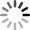Categories

# Create a Loop Function in XtScript

Allright, on the previous post we have know the basic of the basic of Xtgem’s XtScript like variable and print, now on this post we will learn how to create loop function in XtScript.

Creating a loop function in Javascript or PHP is easy because Javascript and PHP has its own loop function which is for, do .. while .., etc.

But XtScript doesn’t have a direct loop function but there are particular command specialized for creating a loop function which is goto.

goto is a command in XtScript to jump to certain jump point declared in the script. The jump point here is declared using @ prefix.### How to create loop function in XtScript using goto

#### Create a simple loop for certain times

On the example below we will create a loop function to do print command ten times.

``<!--parser:xtscript-->	var \$number = 0	var \$statement = You are number	# Declare a jump point	@add_number	var \$number = (\$number + 1)	# Here is the if operator	if \$number <= 10		print \$statement \$number <br/>		goto @add_number	endif<!--/parser:xtscript-->``
You are number 1
You are number 2
You are number 3
You are number 4
You are number 5
You are number 6
You are number 7
You are number 8
You are number 9
You are number 10

Explanation:
On the example above we create an if operator with condition \$number less than or equals to ten. If \$number variable is less than or equals to 10, the script will do print command like above, then jump to @add_number jump point.

At the @add_number jump point, we assign the \$number to be added + 1. And so on after the operation at @add_number jump point is executed, it is reached the if operation again will repeat the same process as before as long as the \$number variable value is less than or equals to 10.

#### Create a loop and print only odd numbers

``<!--parser:xtscript-->	var \$number = 20	print <b>Odd numbers group:</b> <br/>	# Declare a jump point for odd number operation	@odd_number	if \$number == 0		# If the number reaches 0, don't do anythinng but go to @end_loop		goto @end_loop		# Use modulus (%) to decide the number is even or odd	elseif (\$number % 2) != 0		print Found odd number: \$number <br/>		# Subtract the \$number then go to @odd_number to loop this if operation		var \$number = (\$number - 1)		goto @odd_number	else		# If \$number is not 0 and not an odd number, then don't print anything but subtract \$number then jump to @odd_number		var \$number = (\$number - 1)		goto @odd_number	endif	@end_loop	print <em>Odd numbers operation complete</em><!--/parser:xtscript-->``
Odd numbers group:
Found odd number: 19
Found odd number: 17
Found odd number: 15
Found odd number: 13
Found odd number: 11
Found odd number: 9
Found odd number: 7
Found odd number: 5
Found odd number: 3
Found odd number: 1
Odd numbers operation complete

Explanation:
The first argument on the conditional operation above is if \$number == 0. If the \$number is reaching 0, then we go to end this if operation and go to @end_loop

If \$number still more than zero, then we enter the elseif (\$number % 2) != 0. Here we are using modulus % to determine the arithmetic operation with 2. If \$number % 2 result is not equals to 0, it means it is an odd number. If this argument is true, then the commands for this arguments in the code above will be executed then we go to @odd_number to repeat this if operation again.

else the number still more than 0, and yet \$number % 2 equals to 0, we only subtract the \$number variable then go to @odd_number to repeat this if operation again.## By SiDar

Webmaster Assistant and Editor at Indotechboy.com.

SiDar enjoying himself listening J-pop genre music and playing games, especially old school games. Sometimes he also found watching anime and reading manga are interesting when his fellow author recommend him some good titles.